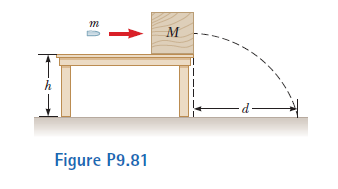# Problem: A bullet of mass m = 8.00 g is fired into a block of mass M = 250 g that is initially at rest at the edge of a table of height h = 1.00 m. The bullet remains in the block, and after the impact the block lands d = 2.00 m from the bottom of the table. Determine the initial speed of the bullet.

98% (27 ratings)
###### Problem Details

A bullet of mass m = 8.00 g is fired into a block of mass M = 250 g that is initially at rest at the edge of a table of height h = 1.00 m. The bullet remains in the block, and after the impact the block lands d = 2.00 m from the bottom of the table. Determine the initial speed of the bullet.Frequently Asked Questions

What scientific concept do you need to know in order to solve this problem?

Our tutors have indicated that to solve this problem you will need to apply the Collisions & Motion (Momentum & Energy) concept. You can view video lessons to learn Collisions & Motion (Momentum & Energy). Or if you need more Collisions & Motion (Momentum & Energy) practice, you can also practice Collisions & Motion (Momentum & Energy) practice problems.

How long does this problem take to solve?

Our expert Physics tutor, Julia took 5 minutes and 6 seconds to solve this problem. You can follow their steps in the video explanation above.

What professor is this problem relevant for?

Based on our data, we think this problem is relevant for Professor Khondaker's class at UCF.

What textbook is this problem found in?

Our data indicates that this problem or a close variation was asked in Physics for Scientists and Engineers - Serway Calc 9th Edition. You can also practice Physics for Scientists and Engineers - Serway Calc 9th Edition practice problems.